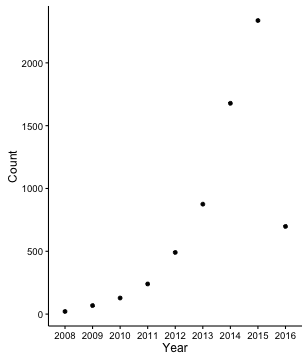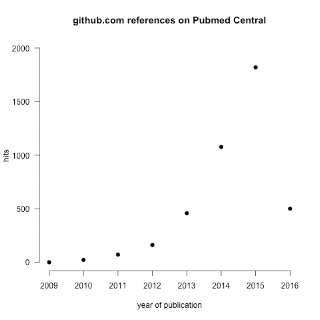## Sunday, 3 April 2016

### GitHub in Numbers for Bioinformaticians

Impact of Github in Numbers:

Total Number of Bioinformatics repositories in Github:Figure 2: Number of Bioinformatics Repositories created by Years (source Github).

Total Number of Bioinformatics Projects by Programming Language:Figure 3: Number of GitHub repositories created in Years by Language (source GitHub)

Number of Forks by Language:Figure 4: Distribution of Number of Forks of Bioinformatics Repositories by Language (source GitHub)

Some of the most popular repositories by Forks:

 Repo Language Forks Watchers Created bigdatagenomics/adam Scala 154 399 2013 chapmanb/bcbb Python 146 298 2008 igvteam/igv Java 81 167 2012 bionode/bionode JavaScript 23 161 2014 tumblr/genesis Ruby 15 133 2014 griffithlab/rnaseq_tutorial R 69 116 2014

Number of Open Issues by Programming Language:Figure 5: Distribution of Number of Open Issues of Bioinformatics Repositories by Language (source GitHub)

Number of references of GitHub.com in PubMed Central:Figure 6: Number of Bioinformatics GitHub mentions in PubMed Central Repositories created by Years (source PubMed Central).

Methods

All numbers where generated with the current R script (replace token with Github API token):

library(devtools)
library(RCurl)
library(RJSONIO)
library(ggplot2)
library(ttutils)
ghSearchRepo <- function(user = NULL, language = "R", only.fullname = TRUE, queryString = NULL, pageSizeNumber = 100){
if(!is.null(user)){
user <- paste0("+user:", user)

}
if(!is.null(language)){
language <- paste0("+language:", language)
}
if(!is.null(queryString)){
query <- paste0(queryString)
}
pageSize <- paste0("&per_page=", pageSizeNumber)
search <- paste0("https://api.github.com/search/repositories?q=",
query,
user,
language,
pageSize,
"&access_token=token")
response <- getURL(search, ssl.verifypeer = FALSE, useragent = "RCurl")
output <- fromJSON(response)
if(output[["total_count"]] == 0){
return(NULL)
}
items <- output\$items;
if(output[["total_count"]] > pageSizeNumber){
numPage = (as.numeric(output[["total_count"]])/as.numeric(pageSizeNumber))+1

for(i in 1:numPage){
page <- paste0("&page=", i)
search <- paste0("https://api.github.com/search/repositories?q=",
query,
user,
language,
pageSize,
page,
"&access_token=token")
response <- getURL(search, ssl.verifypeer = FALSE, useragent = "RCurl")
currentItems <- fromJSON(response)
if(!is.null(currentItems)){
items <- append(items, currentItems\$items)
}
}
}
items
}

addToDF <- function(df, repoList){
length(repoList)
for(i in 1:length(repoList)){
print(repoList[[i]]\$full_name)
repository <- ifelse(is.null(repoList[[i]]\$full_name),"Unknown", repoList[[i]]\$full_name)
language <- ifelse(is.null(repoList[[i]]\$language),"Unknown", repoList[[i]]\$language)
forks_count <- ifelse(is.null(repoList[[i]]\$forks_count),0, repoList[[i]]\$forks_count)
watchers_count <- ifelse(is.null(repoList[[i]]\$watchers_count),0, repoList[[i]]\$watchers_count)
created_at <- ifelse(is.null(repoList[[i]]\$created_at),"Unknown", substr(repoList[[i]]\$created_at,

0,4))
private <- ifelse(is.null(repoList[[i]]\$private),"Unknown", repoList[[i]]\$private)
df <- rbind(df, data.frame("repository" = repository, "language" = language, "forks_count"

= forks_count, "watchers_count" = watchers_count, "created" = created_at, "private" = private))
}
df
}

languageKeywords <- function(df, keyword){

javaRepos = ghSearchRepo(only.fullname = FALSEqueryString = keyword, language = "Java")
df <- addToDF(df, javaRepos)
rRepos = ghSearchRepo(only.fullname = FALSE, queryString = keyword, language = "R")
df <- addToDF(df, repoList = rRepos)
pRepos = ghSearchRepo(only.fullname = FALSE, queryString = keyword, language = "Python")
df <- addToDF(df, repoList = pRepos)
cplusRepos = ghSearchRepo(only.fullname = FALSE, queryString = keyword, language = "C++")
df <- addToDF(df, repoList = cplusRepos)
sRepos = ghSearchRepo(only.fullname = FALSE, queryString = keyword, language = "Scala")
df <- addToDF(df, repoList = sRepos)
jsRepos = ghSearchRepo(only.fullname = FALSE, queryString = keyword, language = "JavaScript")
df <- addToDF(df, repoList = jsRepos)
prRepos = ghSearchRepo(only.fullname = FALSE, queryString = keyword, language = "Perl")
df <- addToDF(df, repoList = prRepos)
shelRepos = ghSearchRepo(only.fullname = FALSE, queryString = keyword, language = "Shell")
df <- addToDF(df, repoList = shelRepos)
rubyRepos = ghSearchRepo(only.fullname = FALSE, queryString = keyword, language = "Ruby")
df <- addToDF(df, repoList = rubyRepos)
cRepos = ghSearchRepo(only.fullname = FALSE, queryString = keyword, language = "C")
df <- addToDF(df, repoList = cRepos)
df
}

df <- data.frame("repository" = character(), "language" = character(), "forks_count" = numeric(),

"watchers_count" = numeric(), "created" = character(),"private"= character())

df <- languageKeywords(df, "bioinformatics")
df <- languageKeywords(df, "genomics")
df <- languageKeywords(df, "proteomics")
df <- languageKeywords(df, "proteins")
df <- languageKeywords(df, "genes")
df <- languageKeywords(df, "spectrometry")
df <- languageKeywords(df, "biology")
df <- unique(df)
df <- subset(df, repository != "Unknown")

Code for pubMed Central by Stephen Eglen:

``````require(devtools)
install_github("njahn82/rebi")

require(rebi)

counts = function(year) {
query = sprintf("github.com AND PUB_YEAR:%d", year)
print(query)
my_data <- epmc_search(query = query)
my_data\$hit_count
}

years = 2009:2015
hits = sapply(years, counts)

png(file="github-usage.png")
plot(years, hits, log='', pch=19, bty='n',
xlab='year of publication',
ylim=c(0, 2000),
las=1, main='github.com references on Pubmed Central')
dev.off()``````